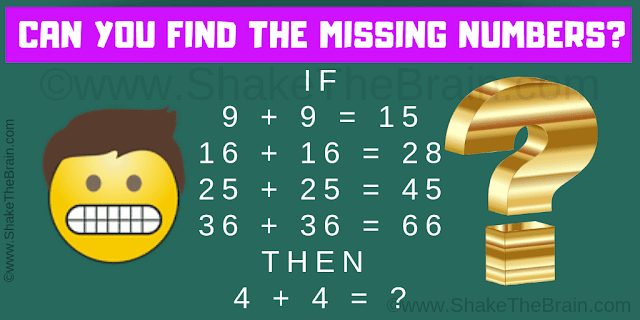## Tuesday, October 29, 2019

Time to put your logical reasoning to test. Here is the picture puzzle which will test your logical reasoning. In this picture puzzle, there are some logical number equations. These equations are incorrect Mathematically but these equations are correct logically. Try to find the logic of these equations and then solve the last equation.Can you solve this Mind Game Math Puzzle?
The answer can be viewed by clicking on the button. Please do give your best try before looking at the answer.

#### 2 comments:

1.2.maybe some of these exist somewhere without published answers. they are incredibly easy though. the pattern is too easy to find in linear equations. thank you for your time.

It will be great to have your comments about this post.